# R S Aggarwal Solutions for Class 11 Maths Chapter 30 Statistics Exercise 30A

Class 11 Chapter 30 – Statistics exercise 30A solutions are provided here. All questions listed in the textbook are solved by subject experts at BYJU’S using step by step format to help the students understand the concepts in an easy way. RS Aggarwal Solutions for Class 11 are a major source of reference material for the students to make their exam preparation more effective. In this section, students will learn to find the mean deviation of the given data.

Class 11 Maths Chapter 30 Statistics Exercise 30A is based on the following topics:

• Mean Deviation (MD)
• Mean Deviation about the mean
• Mean Deviation about the median
• Mean Deviation for ungrouped data
• Mean Deviation for discrete frequency distribution
• Mean Deviation about the mean and median for continuous frequency distribution

Important Formulae: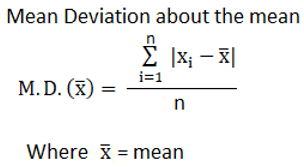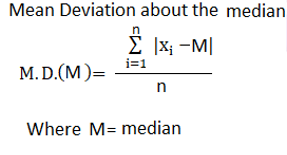## Download PDF of R S Aggarwal Solutions for Class 11 Maths Chapter 30 Statistics Exercise 30A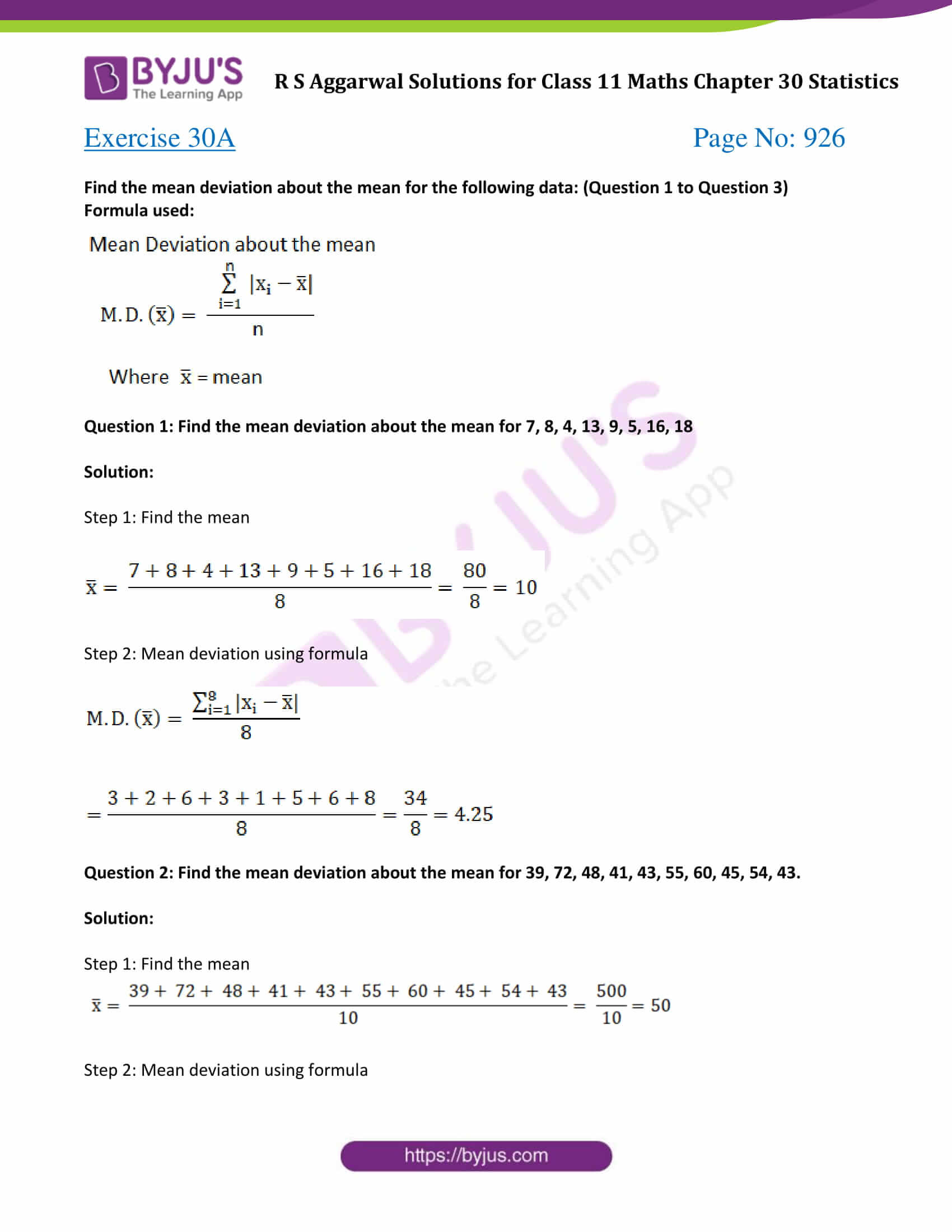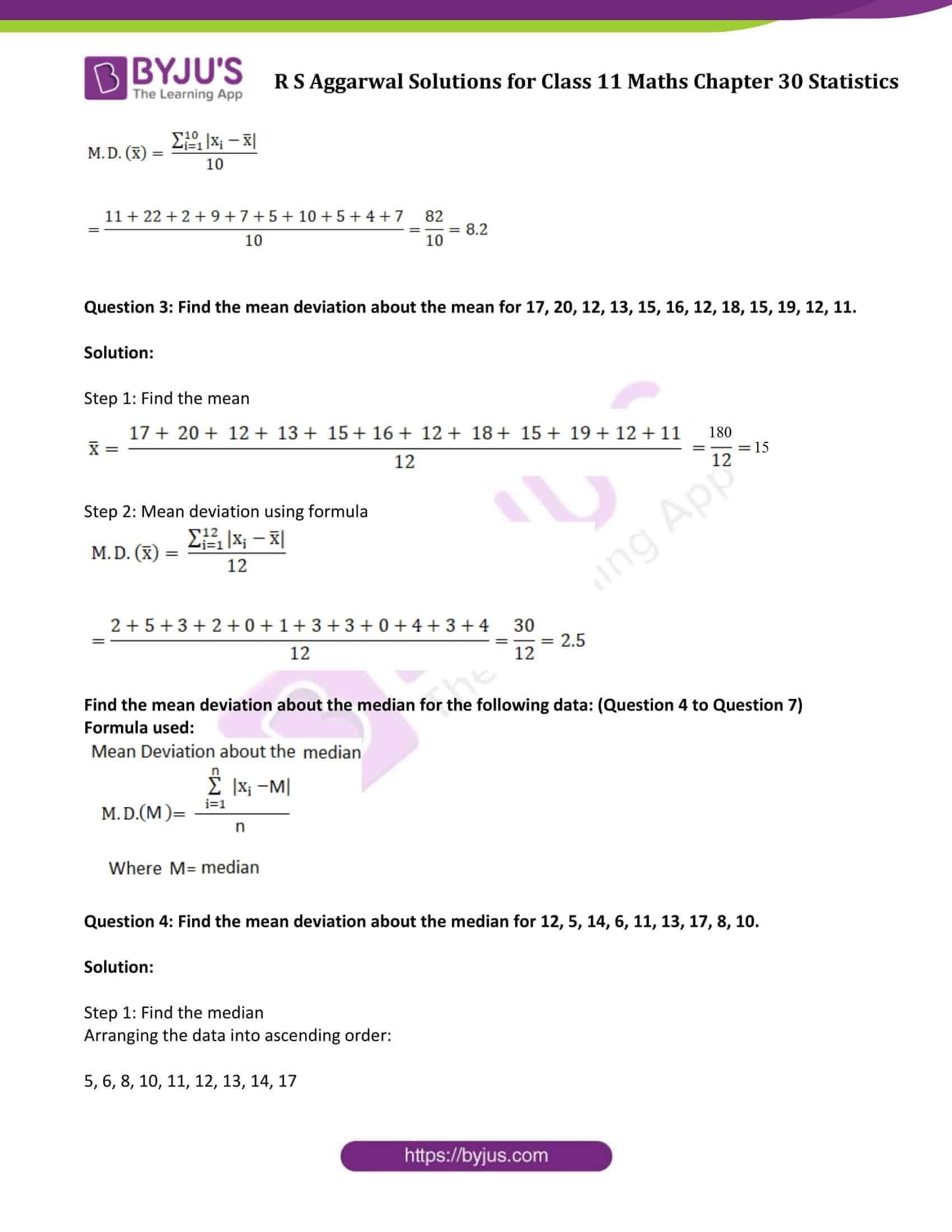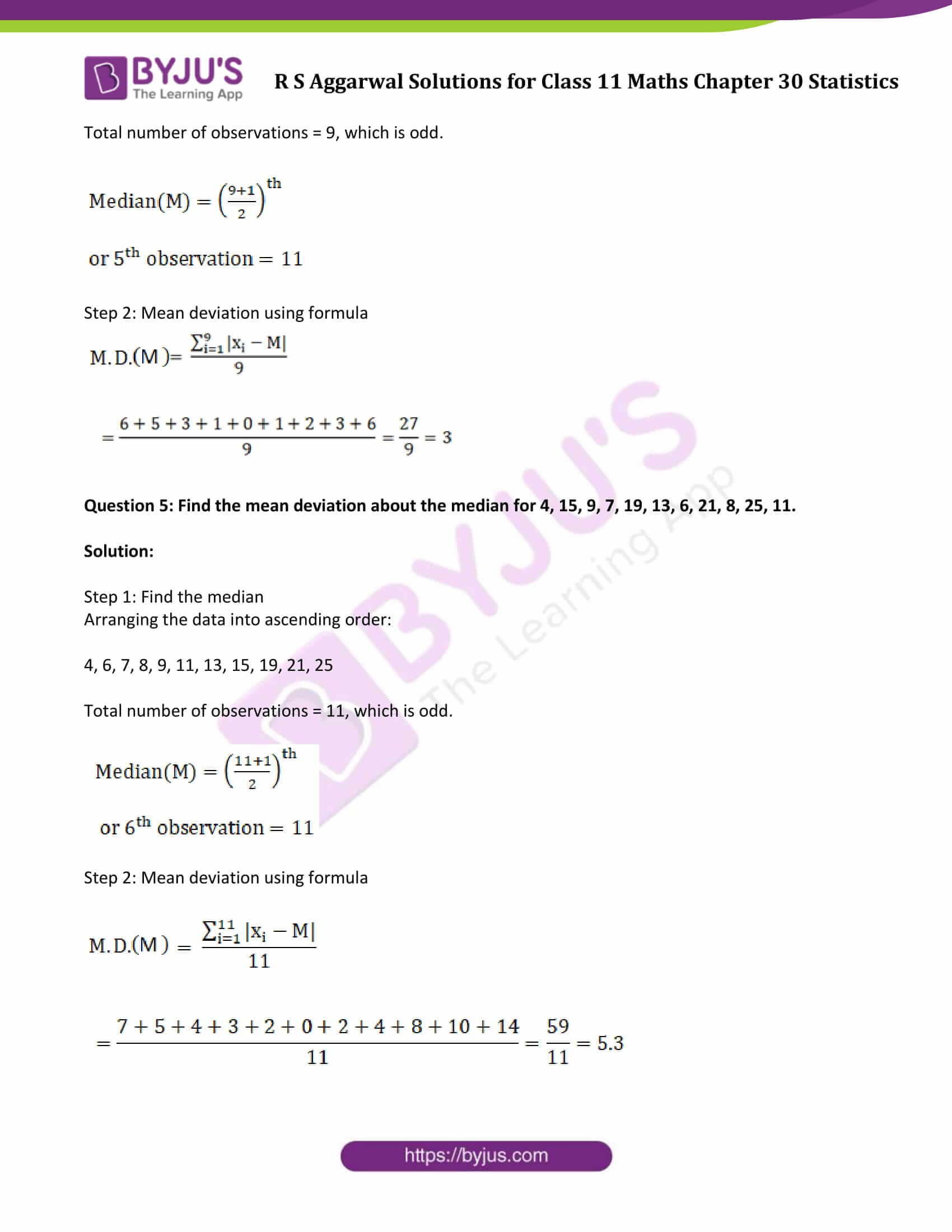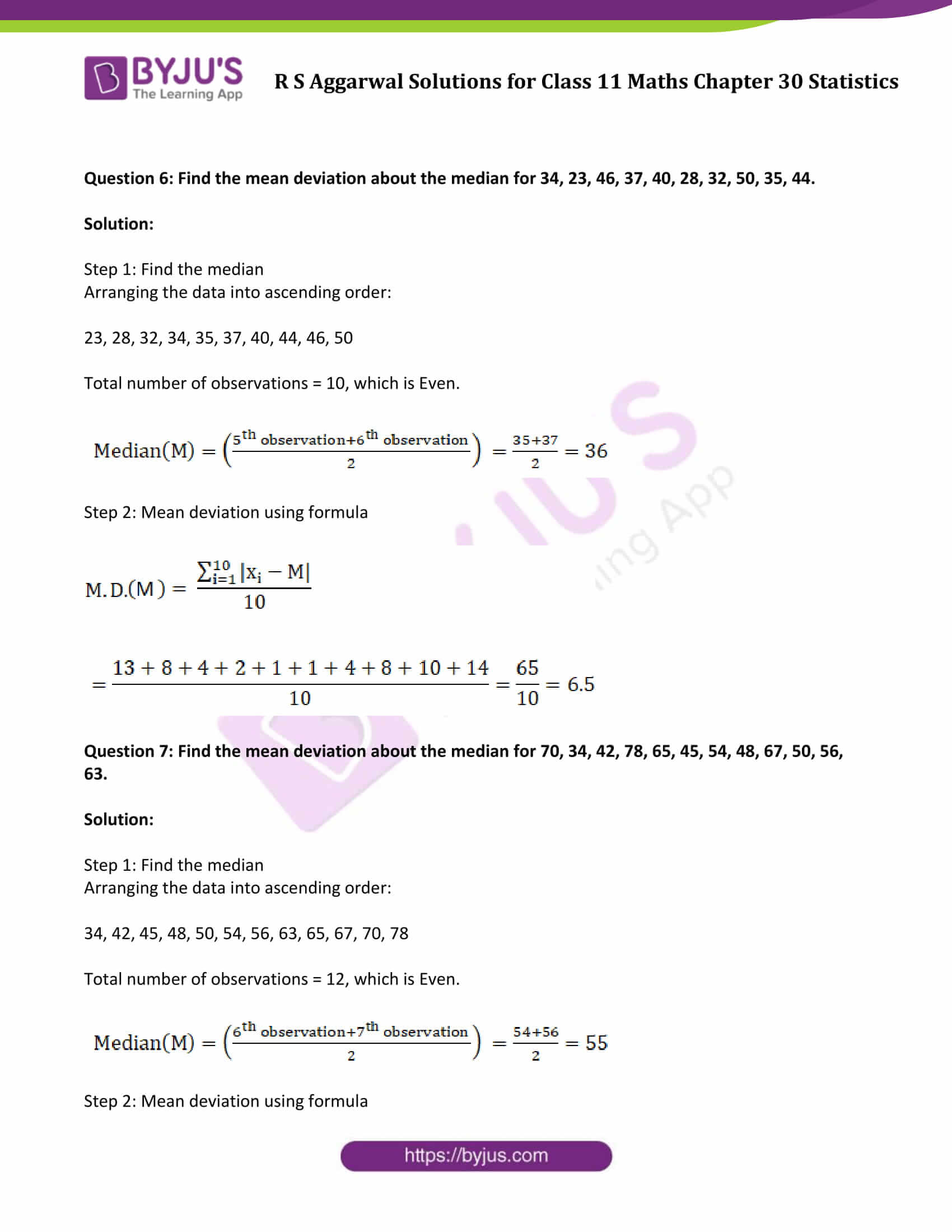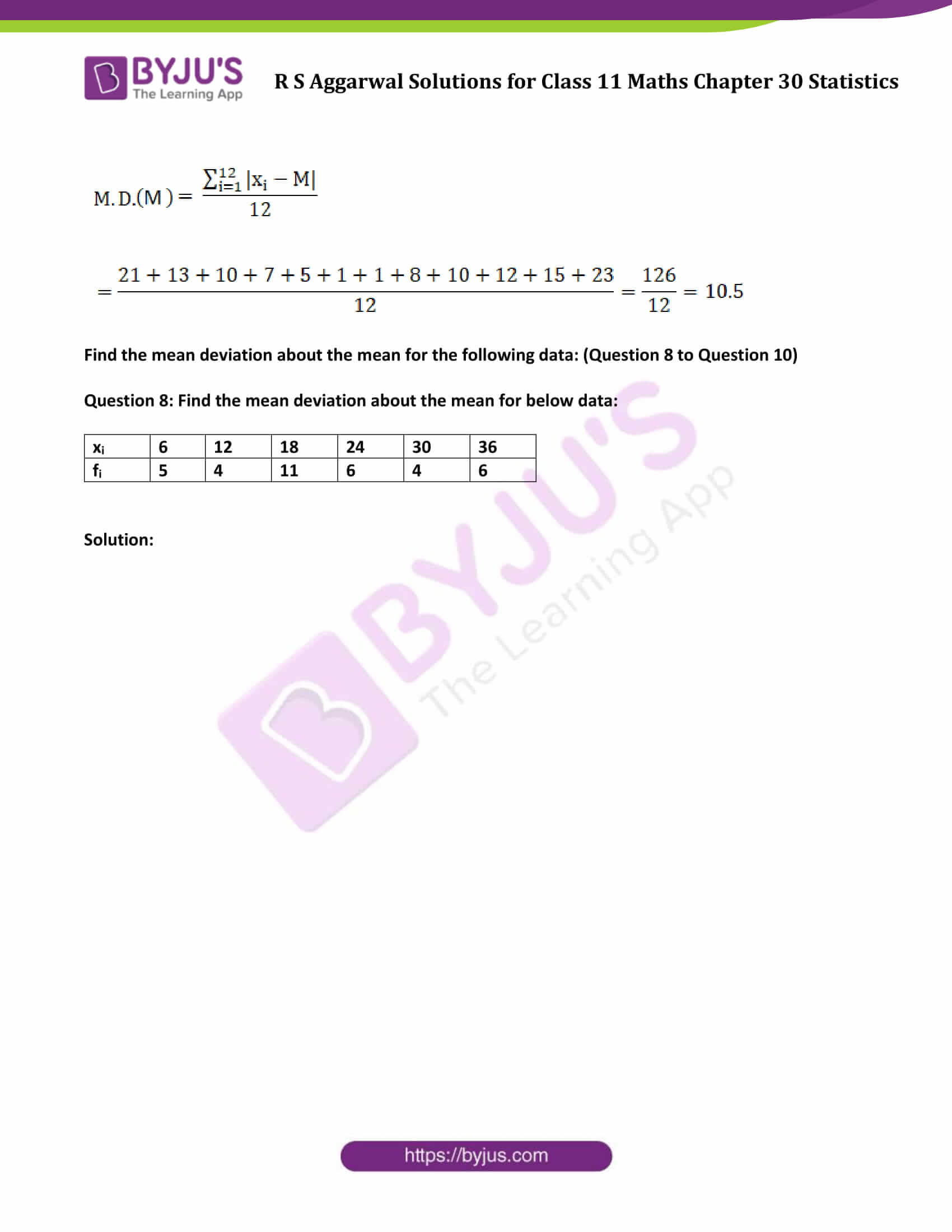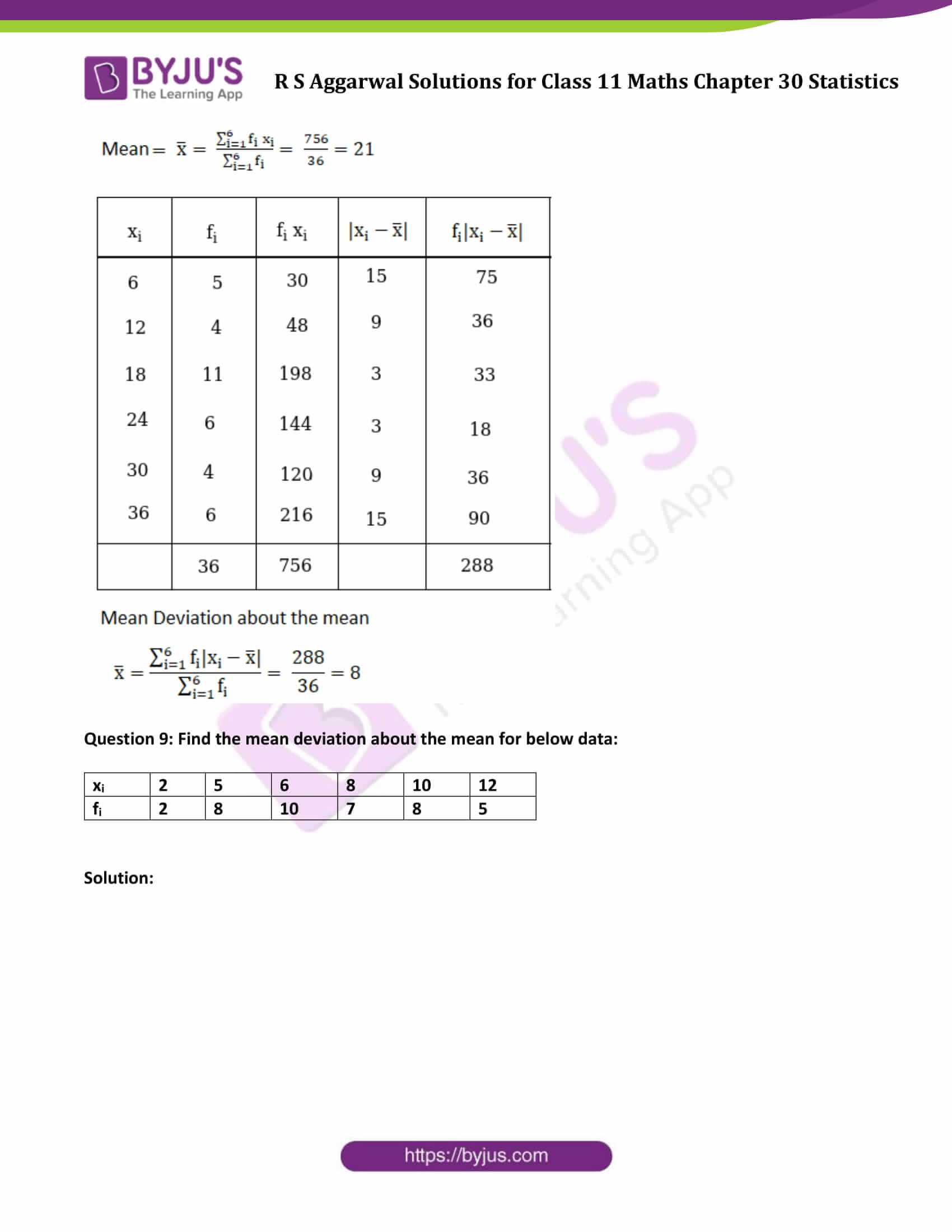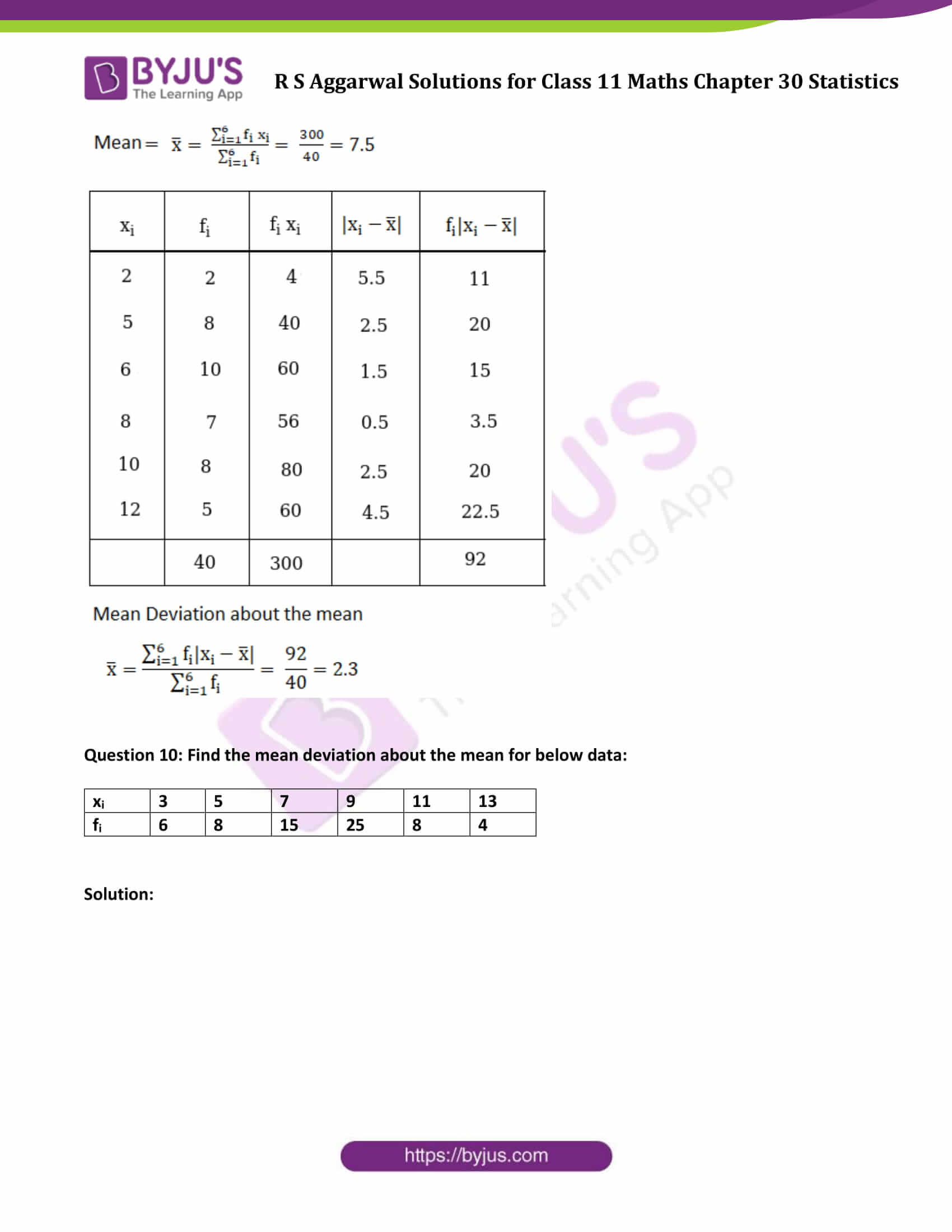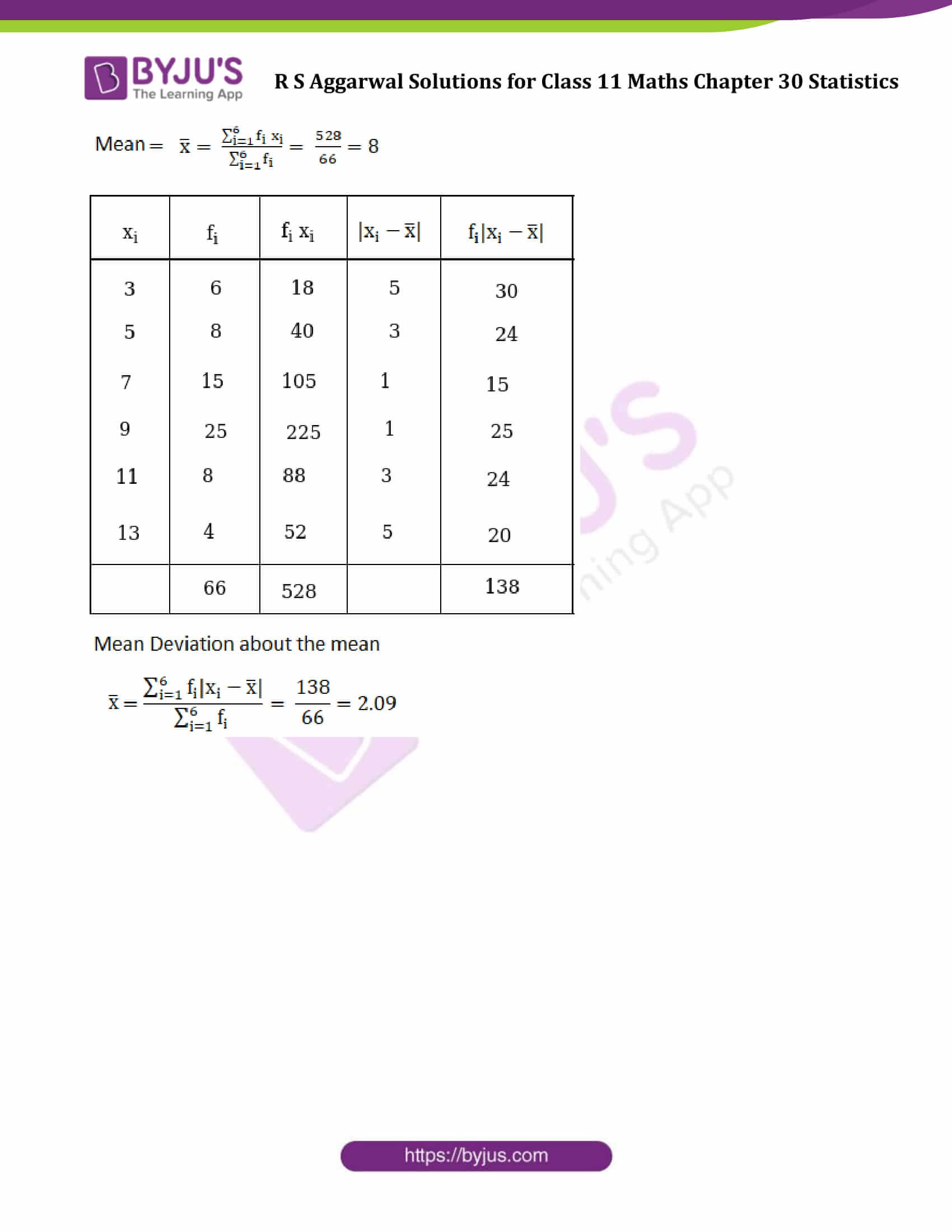### Access Answers to Maths R S Aggarwal Class 11 Chapter 30 Statistics Exercise 30A Page number 926

Find the mean deviation about the mean for the following data: (Question 1 to Question 3)

Formula used: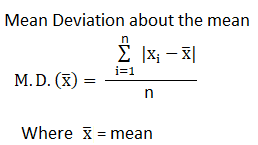Question 1: Find the mean deviation about the mean for 7, 8, 4, 13, 9, 5, 16, 18

Solution:

Step 1: Find the mean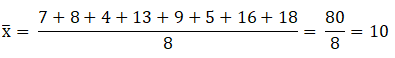Step 2: Mean deviation using formula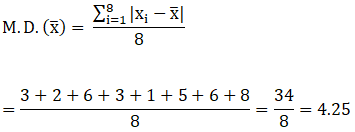Question 2: Find the mean deviation about the mean for 39, 72, 48, 41, 43, 55, 60, 45, 54, 43.

Solution:

Step 1: Find the mean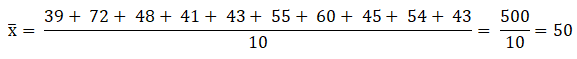Step 2: Mean deviation using formula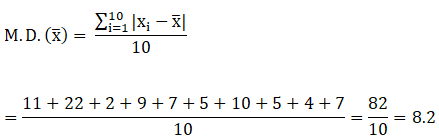Question 3: Find the mean deviation about the mean for 17, 20, 12, 13, 15, 16, 12, 18, 15, 19, 12, 11.

Solution:

Step 1: Find the mean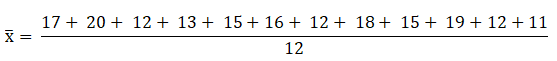= 180/12 = 15

Step 2: Mean deviation using formula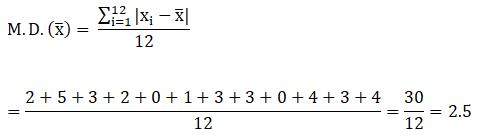Find the mean deviation about the median for the following data: (Question 4 to Question 7)

Formula used: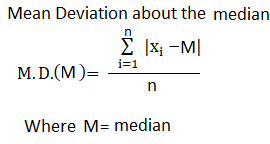Question 4: Find the mean deviation about the median for 12, 5, 14, 6, 11, 13, 17, 8, 10.

Solution:

Step 1: Find the median

Arranging the data into ascending order:

5, 6, 8, 10, 11, 12, 13, 14, 17

Total number of observations = 9, which is odd.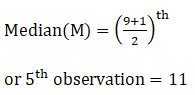Step 2: Mean deviation using formula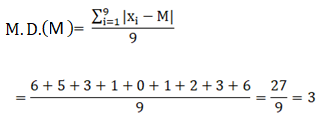Question 5: Find the mean deviation about the median for 4, 15, 9, 7, 19, 13, 6, 21, 8, 25, 11.

Solution:

Step 1: Find the median

Arranging the data into ascending order:

4, 6, 7, 8, 9, 11, 13, 15, 19, 21, 25

Total number of observations = 11, which is odd.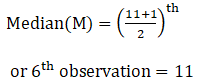Step 2: Mean deviation using formula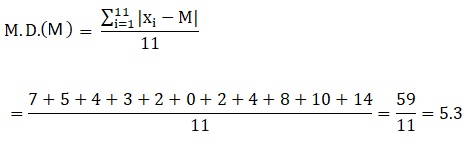Question 6: Find the mean deviation about the median for 34, 23, 46, 37, 40, 28, 32, 50, 35, 44.

Solution:

Step 1: Find the median

Arranging the data into ascending order:

23, 28, 32, 34, 35, 37, 40, 44, 46, 50

Total number of observations = 10, which is Even.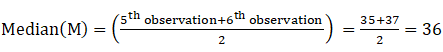Step 2: Mean deviation using formula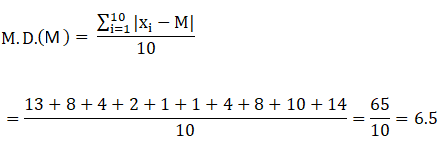Question 7: Find the mean deviation about the median for 70, 34, 42, 78, 65, 45, 54, 48, 67, 50, 56, 63.

Solution:

Step 1: Find the median

Arranging the data into ascending order:

34, 42, 45, 48, 50, 54, 56, 63, 65, 67, 70, 78

Total number of observations = 12, which is Even.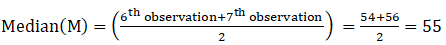Step 2: Mean deviation using formula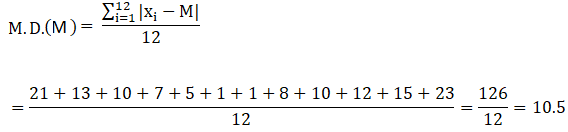Find the mean deviation about the mean for the following data: (Question 8 to Question 10)

Question 8: Find the mean deviation about the mean for below data:

 xi 6 12 18 24 30 36 fi 5 4 11 6 4 6

Solution: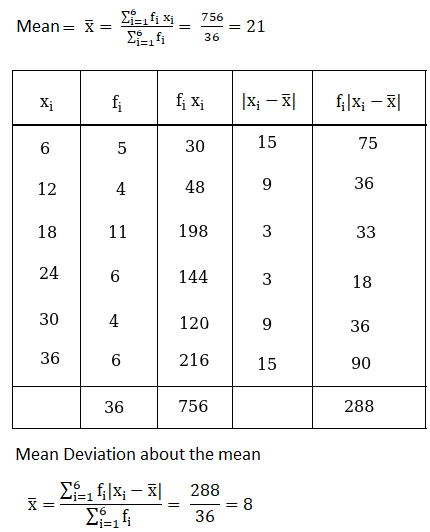Question 9: Find the mean deviation about the mean for below data:

 xi 2 5 6 8 10 12 fi 2 8 10 7 8 5

Solution:Question 10: Find the mean deviation about the mean for below data:

 xi 3 5 7 9 11 13 fi 6 8 15 25 8 4

Solution: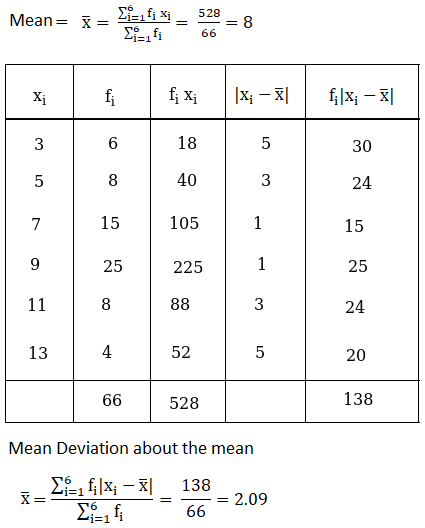## Access other exercise solutions of Class 11 Maths Chapter 30 Statistics

Exercise 30B Solutions

Exercise 30C Solutions

Exercise 30D Solutions